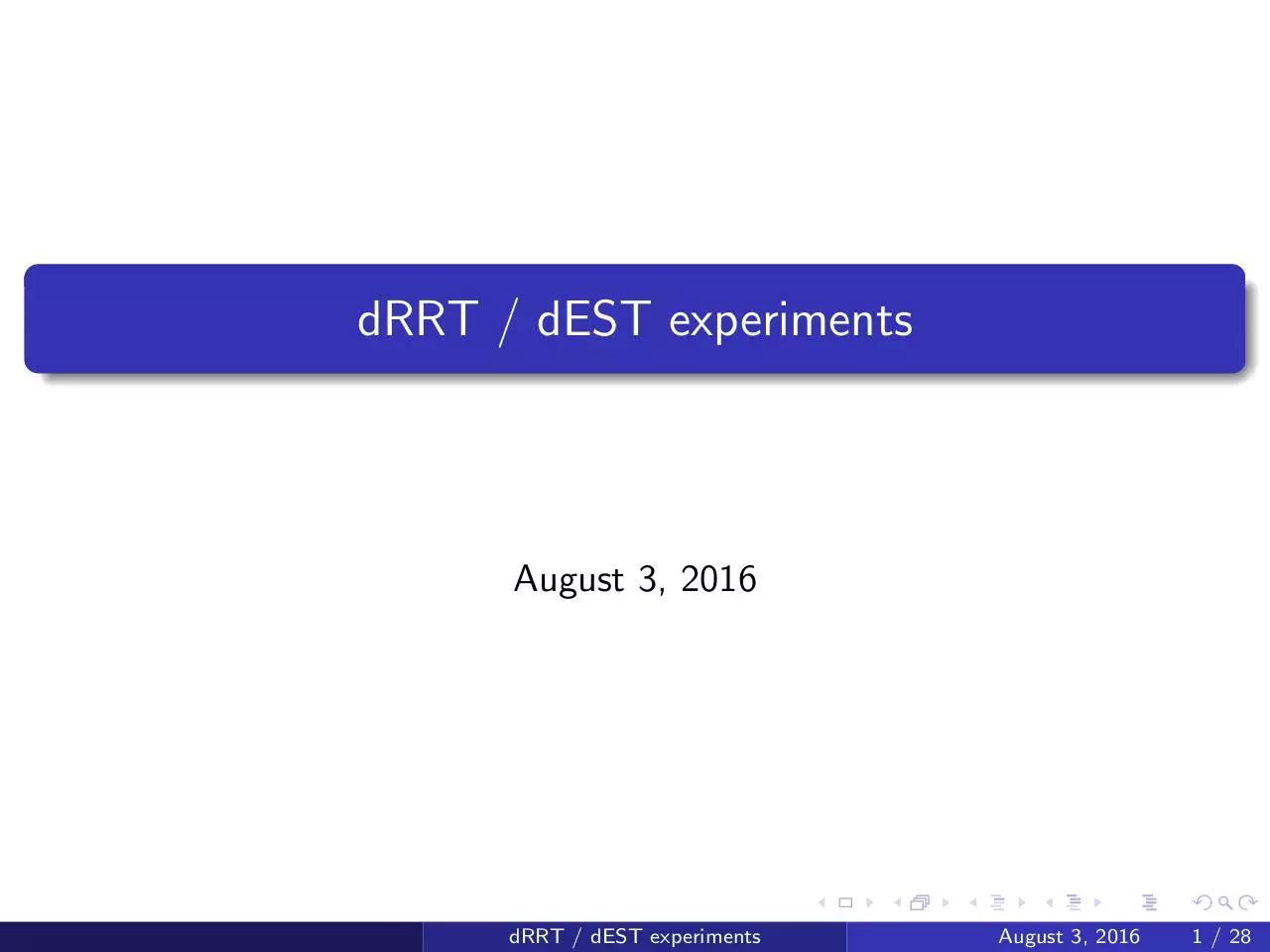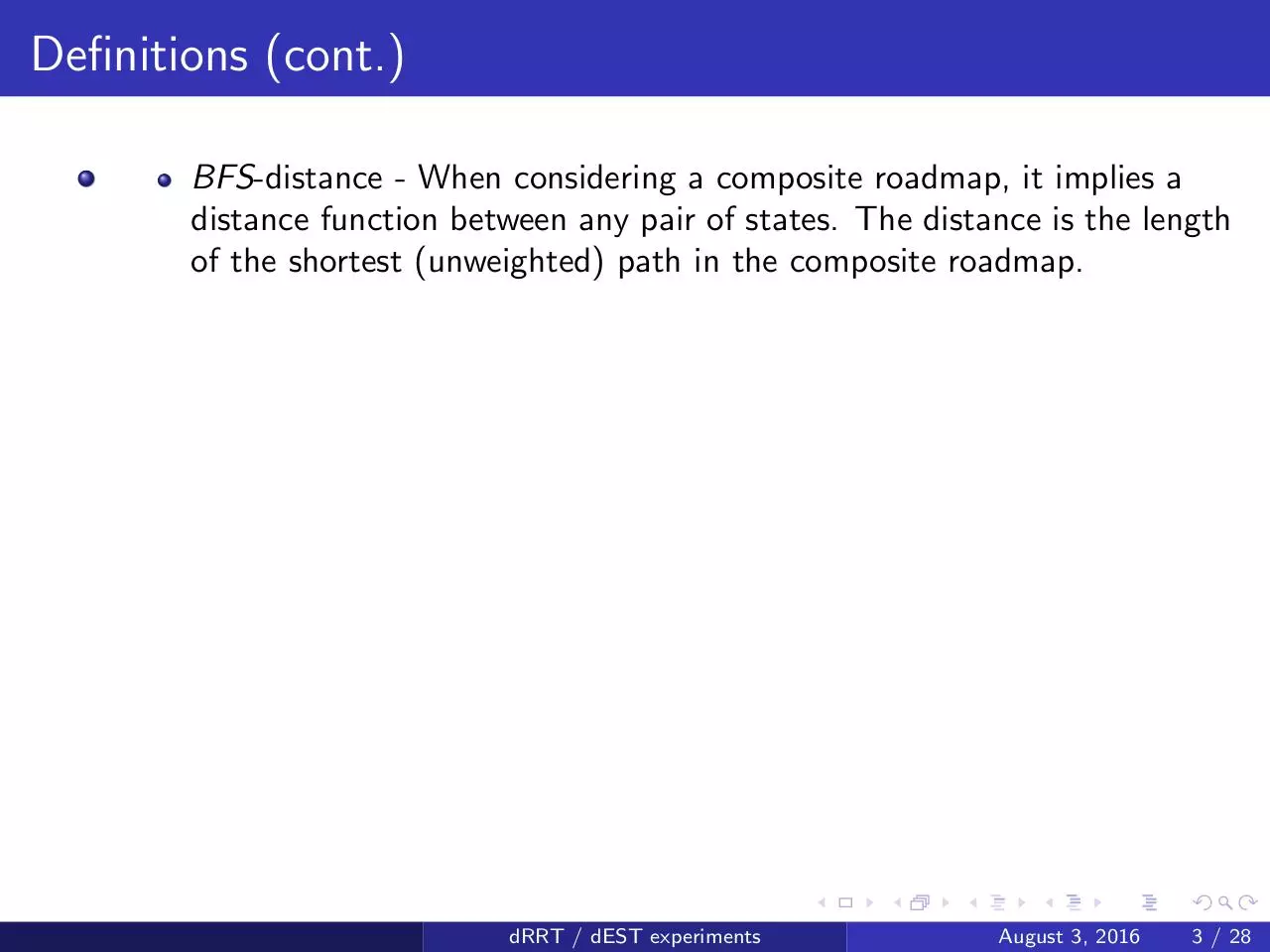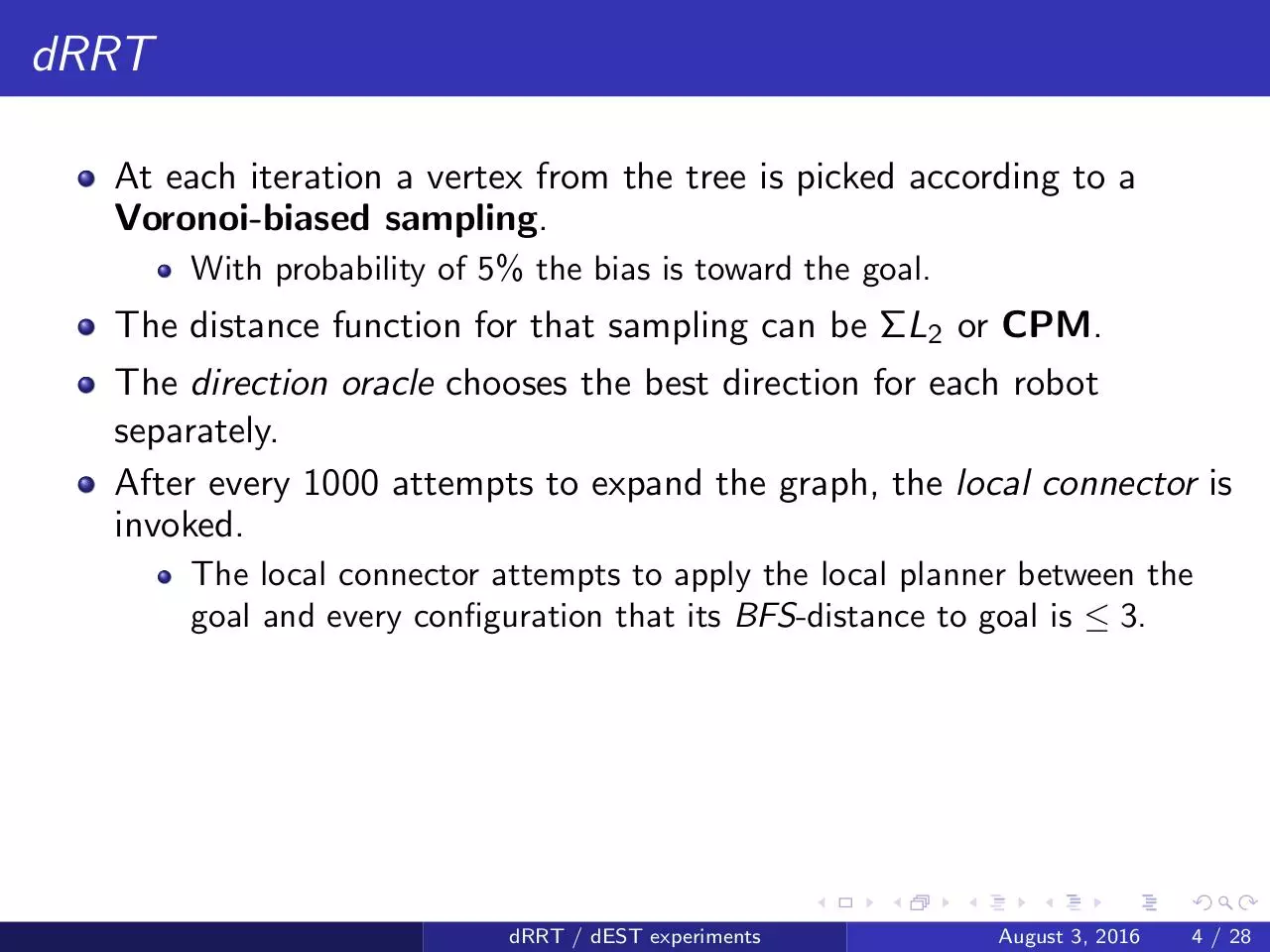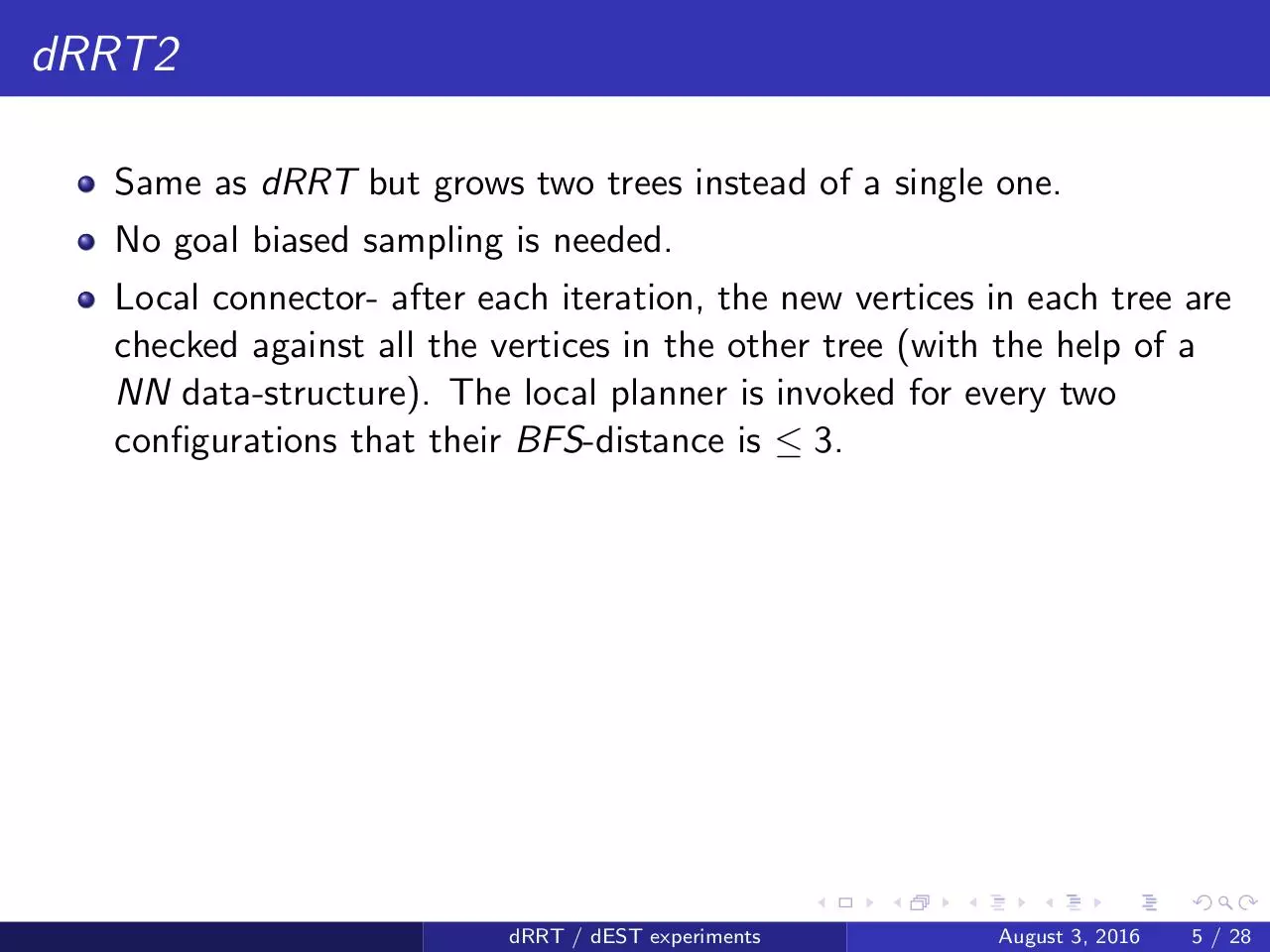# dRRT ex .pdf

### File information

Title: dRRT / dEST experiments

This PDF 1.5 document has been generated by LaTeX with Beamer class version 3.36 / pdfTeX-1.40.16, and has been sent on pdf-archive.com on 03/08/2016 at 07:42, from IP address 192.114.x.x. The current document download page has been viewed 462 times.
File size: 3.29 MB (28 pages).
Privacy: public file

### Document preview

dRRT / dEST experiments

August 3, 2016

dRRT / dEST experiments

August 3, 2016

1 / 28

Definitions
m - The number of robots in the scene.
We assume that the Local Planner connects two configuration by a
straight line and that each robot has a constant speed along it.
Distance functions between two states:
ΣL2 - The sum of L2 distances that each robot travels along the path.
If rotation ispenabled, it is taken with weight 0.5
(L2 (s, t) = ∆x 2 + ∆y 2 + 0.5∆θ).
CPM (Closest Point ”Metric”) Assume translating robots.
Assume that each robot is associated with a radius and a geometric
center (for disk robots this is trivial).
For each pair of robots and configurations s, t we can calculate (in
O(1) time) the time t0 in which their L2 distance is minimal. Let lij
denote that distance.
lij
Let cpmij = ri +r
.
j



Define CPM(s, t) = 100 − min cpmij
i,j

dRRT / dEST experiments

August 3, 2016

2 / 28

Definitions (cont.)
BFS-distance - When considering a composite roadmap, it implies a
distance function between any pair of states. The distance is the length
of the shortest (unweighted) path in the composite roadmap.

dRRT / dEST experiments

August 3, 2016

3 / 28

dRRT
At each iteration a vertex from the tree is picked according to a
Voronoi-biased sampling.
With probability of 5% the bias is toward the goal.

The distance function for that sampling can be ΣL2 or CPM.
The direction oracle chooses the best direction for each robot
separately.
After every 1000 attempts to expand the graph, the local connector is
invoked.
The local connector attempts to apply the local planner between the
goal and every configuration that its BFS-distance to goal is ≤ 3.

dRRT / dEST experiments

August 3, 2016

4 / 28

dRRT2
Same as dRRT but grows two trees instead of a single one.
No goal biased sampling is needed.
Local connector- after each iteration, the new vertices in each tree are
checked against all the vertices in the other tree (with the help of a
NN data-structure). The local planner is invoked for every two
configurations that their BFS-distance is ≤ 3.

dRRT / dEST experiments

August 3, 2016

5 / 28

dEST
Grows two trees.
Maintain a distribution over the vertices of the tree named wpick .
wpick (x) is the inverse of the number of vertices in the tree that are in
the neighborhood of x.
For the neighborhood we can use:
ΣL2 - with radius equals to the farthest point from x among its
neighbors in the composite graph.
CPM- with radius equals to a typical CPM value between two
neighbors in the graph (neighbors are sampled at random at the
beginning of the algorithm, and the median is taken as the radius).

At each iteration a vertex is sampled according to wpick .
K = 5 neighbors of it are uniformly sampled.
For each neighbor, the local planner is invoked with probability that is
proportional to its success rate (estimated with ”machine learning”
techniques based on CPM).
Local connector- same as in dRRT2
dRRT / dEST experiments

August 3, 2016

6 / 28

Tested Robots

dRRT / dEST experiments

August 3, 2016

7 / 28

Easy Environments

(a) 2 robots

(b) 3 robots

(c) 4 robots

(d) 5 robots

Figure: Easy environments with translating robots

dRRT / dEST experiments

August 3, 2016

8 / 28

(a) N = 500, K = 10

(b) N = 1000, K = 2e log N = 37

Figure: A roadmap for a single robot

dRRT / dEST experiments

August 3, 2016

9 / 28dRRT-ex.pdf (PDF, 3.29 MB)

### Share on social networks

#### HTML Code

Copy the following HTML code to share your document on a Website or Blog